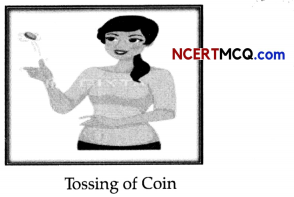Students can access the CBSE Sample Papers for Class 11 Maths with Solutions and marking scheme Term 2 Set 3 will help students in understanding the difficulty level of the exam.

## CBSE Sample Papers for Class 11 Maths Term 2 Set 3 for Practice

Time: 2 Hours
Maximum Marks:40

General Instructions:

• This question paper contains three sections A, B and C. Each part is compulsory.
• Section -A has 6 short answer type (SA1) questions of 2 marks each.
• Section -B has 4 short answer type (SA2) questions of 3 marks each.
• Section -C has 4 long answer type questions (LA) of 4 marls each.
• There is an internal choice in some of the questions.
• Q14 is a case-based problem having 2 sub parts of 2 marks each.

Section – A

Question 1.
Write the radian measure of 5°37’30”. (2)

Question 2.
Solve $$\frac{1}{x-2}$$ < 0, x ∈ R. (2)
OR
Solve, 0 < $$\frac{-x}{3}$$ < 1, x ∈ R.Question 3.
A polygon has 35 diagonals. Find the number of its sides. (2)

Question 4.
If the distance between the points (a, 2,1) and (1, -1, 1) is 5 units, then find the value(s) of a (2)

Question 5.
Find f'(x), if f(x) = (x – 2)2 (2x – 3). (2)

Question 6.
4 cards are drawn from a well-shuffled deck of 52 cards. What is the probability of obtaining 3 diamonds and one spade ? (2)

Section – B

Question 7.
Show that, if x2 + y2 = 1, then the point (x, y, $$\sqrt{1-x^{2}-y^{2}}$$) is at a distance 1 unit from the origin.
OR
Show that the point A( 1, -1, 3), B(2, -4, 5) and C(5, -13, 11) are collinear. (3)

Question 8.
A group consists of 4 girls and 7 boys. In how many ways can a team of 5 members be selected, if the
team has (3)
(i) no girls.
(ii) at least one boy and one girl.
(iii) at least three girls.
OSWAAL CBSE Sample Question Papers Term-ll, MATHEMATICS, Class-XI

Question 9.
Prove that: $$\frac{\cos x}{1-\sin x}$$ = tan $$\left(\frac{\pi}{4}+\frac{x}{2}\right)$$
OR
Prove that:
sinx + sin 2x + sin 4x + sin 5x = 4cos $$\frac{x}{2}$$ cos $$\frac{3x}{2}$$ sin 3x (3)

Question 10.
If tan A = $$\frac{a}{a+1}$$ and tan B = $$\frac{1}{2 a+1}$$, then find the value of A + B. (3)

Section – C

Question 11.
Solve the following inequality graphically in two-dimensional plane:
x + y < 5 (4)

Question 12.
Find the equation of the hyperbola whose conjugate axis is 5 and distance between the foci is 13.
OR
Find the equation of the hyperbola passing through the point (2, 3) and having foci (0, ± √10). (4)

Question 13.
Find the derivative of the $$\frac{x}{\sin ^{n} x}$$ function where n is the non zero constant (4)Case-Based/Data Based

Question 14.
In a game, the entry fee is ₹ 5. The game consists of a tossing a coin 3 times, If one or two heads show, Sweta gets her entry fee back. If she throws 3 heads, she receives double entry fees. Otherwise she will lose. For tossing a coin three times, find the following:Based on above information, answer the following question.
(i) Find the Probability that she loses the entry fee.
(ii) Find the Probability that she just gets her entry fee and also find the Probability that she gets double entry fee.